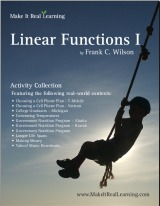August SALE! Get Math Mammoth and Make It Real Learning downloads & CDs at 25% off — right here at the MathMammoth.com website. Use coupon code V827-VMC3-HK7V at checkout. Offer valid through August 25, 2022.

-->
^
You are here: HomeMake It Real Learning → Linear Functions I

# Make It Real Learning Linear Functions I workbook46 pages
(10 activities)

Free Sample (PDF):
Choosing a Cell Phone Plan (Verizon)

# Introduction

The Make It Real Learning Linear Functions I workbook focuses on real-world situations that may be effectively modeled by linear equations. This book is suitable for both algebra 1 and algebra 2 students.

Whether choosing a cell phone plan or downloading music, learners will discover how to use linear functions to help them become better informed consumers. Each activity integrates real world information, companies, and issues (e.g. Yahoo!, T-Mobile, life spans, WIC, making money), not just "realistic" data.

There are multiple ways to use the activities of the book in a teaching environment. The activities are an excellent tool for stimulating mathematical discussions in a small group setting. Due to the challenging nature of each activity, group members are motivated to brainstorm problem solving strategies together. The interesting real world contexts motivate them to want to solve the problems. The activities may also be used for individual projects and class-wide discussions.

As a ready-resource for teachers, the workbook also includes completely worked-out solutions for each activity. To make it easier for teachers to assess student work, the solutions are included on a duplicate copy of each activity.

## Linear Functions I Activity Objectives

Activity TitleMathematical Objectives
Choosing a Cell Phone Plan - T-Mobile:
Investigating Linear Functions
Create and evaluate linear function models
Solve linear equations
Use quantitative analysis to make a consumer decision
Choosing a Cell Phone Plan - Verizon:
Investigating Linear Equations
Create and evaluate linear function models
Solve linear equations
Use quantitative analysis to make a consumer decision
Using Linear Function Models
Interpret the real-world meaning of the slope of a line
Solve linear equations and graph linear functions
Find the point of intersection of two lines
Converting Temperatures:
Solving Linear Equations
Evaluate a linear function and solve a linear equation
Find the inverse of a linear function
Create formulas to estimate unknown values
Linear Function Modeling
Calculate an average rate of change
Create a linear model
Evaluate a linear function symbolically and graphically
Government Nutrition Program - Hawaii:
Linear Function Modeling
Calculate an average rate of change
Create a linear model
Evaluate a linear function symbolically and graphically
Government Nutrition Program:
Linear Function Modeling
Calculate an average rate of change
Create a linear model
Evaluate a linear function symbolically and graphically
Longer Life Spans:
Using Linear Function Models
Determine what type of function best fits a scatter plot
Use regression to find a linear model
Interpret the practical meaning of the slope of a linear function
Solve a linear equation and interpret the meaning of the result
Making Money:
Working with Direct Proportionality
Create and graph a linear function model
Determine if two quantities are directly proportional
Compose two linear functions
Use numerical results to make informed consumer decisions
Working with Linear Equations
Determine the domain of a function
Create a system of linear equations
Interpret the practical meaning of a point of intersection
Solve a system of equations

Bundle specials!

Activity Library, Volume I. Price: \$39.99
Includes 11 Make It Real Learning activity workbooks for the price of 8! You get three workbooks for free!

Activity Library, Volume II. Price: \$39.99
Includes 11 Make It Real Learning activity workbooks for the price of 8! You get three workbooks for free!

States by the Numbers Bundle. Price: \$19.99
Inludes all 50 States by the Numbers workbooks at a whopping 87% discount!
(Normal price would be 50 × \$2.99 = \$149.50)

By purchasing any of the Make It Real Learning workbooks, permission IS granted for the teacher (or parent) to reproduce this material to be used with his/her students in a teaching situation; not for commercial resale. However, you are not permitted to share the material with another teacher.

In other words, you are permitted to make copies for the students/children you are teaching, but not for other teachers' usage.

WAIT!

Receive my monthly collection of math tips & resources directly in your inbox — and get a FREE Math Mammoth book!You can unsubscribe at any time.

### Math Mammoth Tour

Confused about the different options? Take a virtual email tour around Math Mammoth! You'll receive:

An initial email to download your GIFT of over 400 free worksheets and sample pages from my books. Six other "TOURSTOP" emails that explain the important things and commonly asked questions concerning Math Mammoth curriculum. (Find out the differences between all these different-colored series!)

This way, you'll have time to digest the information over one or two weeks, plus an opportunity to ask me personally about the curriculum.
A monthly collection of math teaching tips & Math Mammoth updates (unsubscribe any time)### "Mini" Math Teaching Course

This is a little "virtual" 2-week course, where you will receive emails on important topics on teaching math, including:

- How to help a student who is behind
- Troubles with word problems
- Teaching multiplication tables
- Why fractions are so difficult
- The value of mistakes
- Should you use timed tests
- And more!

A GIFT of over 400 free worksheets and sample pages from my books right in the very beginning.A monthly collection of math teaching tips & Math Mammoth updates (unsubscribe any time)
Enter your email to receive math teaching tips, resources, Math Mammoth news & sales, humor, and more! I tend to send out these tips about once monthly, near the beginning of the month, but occasionally you may hear from me twice per month (and sometimes less often).• A GIFT of over 400 free worksheets and sample pages from my books.